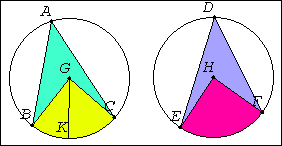# Proposition 27

In equal circles angles standing on equal circumferences equal one another whether they stand at the centers or at the circumferences.

For in equal circles ABC and DEF, on equal circumferences BC and EF, let the angles BGC and EHF stand at the centers G and H, and the angles BAC and EDF at the circumferences.

I say that the angle BGC equals the angle EHF, and the angle BAC equals the angle EDF.I.23

For, if the angle BGC does not equal the angle EHF, one of them is greater. Let the angle BGC be greater. Construct the angle BGK equal to the angle EHF on the straight line BG and at the point G on it.

I.26

Now equal angles stand on equal circumferences when they are at the centers, therefore the circumference BK equals the circumference EF.

But EF equals BC, therefore BK also equals BC, the less equals the greater, which is impossible.

Therefore the angle BGC is not unequal to the angle EHF, therefore it equals it.

III.20

And the angle at A is half of the angle BGC, and the angle at D half of the angle EHF, therefore the angle at A also equals the angle at D.

Therefore in equal circles angles standing on equal circumferences equal one another whether they stand at the centers or at the circumferences.

Q.E.D.

## Guide

This proposition is used in a few propositions in Books III, IV, VI, and XII starting with III.29.